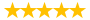# Assume that demand for a commodity is represented by the equation

Question # 00004276 Posted By: expert-mustang Updated on: 11/30/2013 08:03 PM Due on: 12/01/2013
Subject Economics Topic General Economics Tutorials:
Question

Assume that demand for a commodity is represented by the equation P = 10 – 0.2 Qd, and supply by the equation P = 2 + 0.2 Qs where Qd and Q s are quantity demanded and quantity supplied, respectively, and P is the Price. Use the equilibrium condition Qs = Qd

Tutorials for this Question
1. ## Solution: Assume that demand for a commodity is represented by the equation

Tutorial # 00004075 Posted By: expert-mustang Posted on: 11/30/2013 08:05 PM
Puchased By: 3
Tutorial Preview
The solution of Assume that demand for a commodity is represented by the equation...
Attachments
Demand_n_Supply_Explanatin.docx (26.9 KB)
Recent Feedback
Rated By Feedback Comments Rated On
c...cheAppreciate the hard work of the team 03/21/2014

Great! We have found the solution of this question!